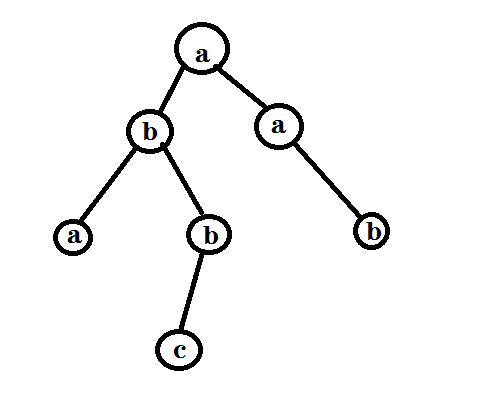Trie树入门

2019-02-18 20:01:32

Trie树入门

PS:本文章所有代码未经编译，有错误还请大家指出。

引入

​ 给定一本字典中的 $n$ 个单词，还有 $m$ 个询问。每次询问询问一个单词是否出现在这 $n$ 个单词中。

暴力

for(int l;m;m--)
{
bool flg=false;
scanf("%s",str);
l=strlen(str);
for(int i=1;i<=n;i++)
{
int len=strlen(s[i])
if(l!=len)continue;
for(int j=1;j<=len;j++)
{
if(s[i][j]!=str[j])
{
flg=true;
break;
}
}
if(flg)
{
for(int j=1;j<=len;j++)
printf("%c",s[i][j]);
}
putchar('\n');
}
}

深入

Q:空间浪费在了哪里？

A:容易发现的是，在保证单词不乱序的情况下，他们的部分前缀是相同的！那么我们是不是可以从这里入手来减少空间复杂度呢？

1. 相同的可以压缩成一个节点(那我们就能将这些前缀压缩来减少空间消耗)
2. 树上路径唯一。(那我们就可以保证每一个单词之间的存储互不影响)

浅谈Trie树

简介

​ Trie是一种多叉树，在对单词处理中是经常利用的一种数据结构，是树结构中一种较为特殊的树结构，它在计算机中对字符采用链表方式存储 。

​ ---百度百科

基本性质

Trie的核心思想是空间换时间，利用字符串的公共前缀来降低查询时间的开销以达到提高效率的目的。

1. 根节点不包含字符，除根节点外每一个节点都只包含一个字符。

2. 从根节点到某一节点，路径上经过的字符连接起来，为该节点对应的字符串。

3. 每个节点的所有子节点包含的字符都不相同。

​ ---百度百科

形态

1. 将字符存储在边上
2. 将字符存储在点上构建

code

void ins(char *s)
{
int rt=0;
for(int i=0,v;s[i];i++)
{
int v=s[i]-'a';
if(!trie[rt][v])
trie[rt][v]=++tot;//给每个节点一个编号,tot为全局变量
rt=trie[rt][v];//向下一个节点,继续存储当前单词
}
}

查询操作

Trie树一般支持两种查询操作：

1. 查询单词是否出现过。
2. 查询单词的出现次数。

code

bool find(char *s)
{
int rt=0;
for(int i=0,v;s[i];i++)
{
v=s[i]-'a';
if(!trie[rt][v])retturn false;
rt=trie[rt][v];
}
if(ok[rt])return true;
//加上这一句可以判断当前单词是否出现过。
//而如果不加，就可以判断当前单词是不是某个单词的前缀
//如果加上这一句的话就在构建的时候对应的在词尾标记即可。
return false;
}

01Trie

实现

01字典树的实现可以看成是把一个数的二进制字符化后插入到一棵一般的字典树中。 那你们会不会有疑问就是说 当某一个数的二进制位是另一个数的二进制位的某一前缀的时候 是不是查不到这个数 $$7 =111{(2)} \3=11{(2)}$$ 然后这个时候 我们可以做一个处理 即 从一个特别特别长的位置开始插入(一般会选择 1<<30位开始处理 ，对应：01字典树种插入3时 相当于在字典树中插入00 …..00011) 但还是要见题而异

构建

code

void ins(int x)
{
int rt=0;
for(int i=1<<30;i;i>>=1)
{
bool c=x&i;
//这里推荐写bool类型，不推荐写int类型，因为很容易忘记是对应位为1
if(!trie[rt][c])
trie[rt][c]=++tot;//记录节点编号
rt=trie[rt][c];
}
}

查询最大异或和

code

int query(int x)
{
int ans=0,rt=0;
for(int i=1<<30;i;i>>=1)
{
bool c=x&i;
if(trie[rt][c^1])
ans+=i,rt=trie[rt][c^1];
//如果有我们就选择这个较高位来实现我们的贪心策略.记得累计答案
else rt=trie[rt][c];
}
return ans;
}

• star
首页